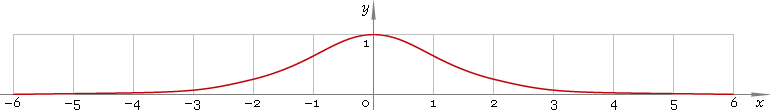The Art of Interface

# sech — hyperbolic secant function

Category. Mathematics.

Abstract. Hyperbolic secant: definition, graph, properties and identities.

## 1. Definition

Hyperbolic secant is defined as

sechx ≡ 2 /(ex + ex)

## 2. Graph

Hyperbolic secant is symmetric function defined everywhere on real axis. Its graph is depicted below — fig. 1.Fig. 1. Graph of the hyperbolic secant function y = sechx.

Function codomain is limited to the range (0, 1].

## 3. Identities

Base:

sech2x + tanh2x = 1

By definition:

sechx ≡ 1 /coshx

Property of symmetry:

sech−x = sechx

## 4. Support

Hyperbolic secant function sech is supported in:

Hyperbolic secant function of the complex argument sech is supported in:

## 5. How to use

To calculate hyperbolic secant of the number:

``sech(-1);``

To calculate hyperbolic secant of the current result:

``sech(Rslt);``

To calculate hyperbolic secant of the number x in memory:

``sech(Mem[x]);``